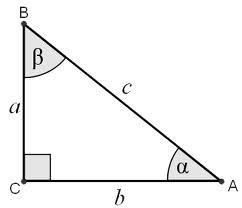# Right-angled 3511

In a right-angled triangle at vertex C, the alpha angle is 24 degrees smaller than the beta angle to determine the size of the triangle angles.

A =  33
B =  57

### Step-by-step explanation:

A=B-24
A+B=90

A-B = -24
A+B = 90

Row 2 - Row 1 → Row 2
A-B = -24
2B = 114

B = 114/2 = 57
A = -24+B = -24+57 = 33

A = 33
B = 57

Our linear equations calculator calculates it.Did you find an error or inaccuracy? Feel free to write us. Thank you!

Tips for related online calculators
Do you have a system of equations and looking for calculator system of linear equations?